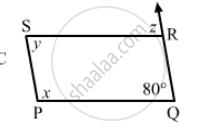Advertisement Remove all ads

# The Following Figure is Parallelogram. Find the Degree Values of the Unknown X, Y, Z. - Mathematics

The following figure is parallelogram. Find the degree values of the unknown xyzAdvertisement Remove all ads

#### Solution

$\text{ Opposite angles are equal in a parallelogram } .$
$\therefore y = 80°$
$y + x = 180°$
$x = 180° - 100°= 80°$
$z = y = 80° (\text{ alternate angles })$

Is there an error in this question or solution?
Advertisement Remove all ads

#### APPEARS IN

RD Sharma Class 8 Maths
Chapter 17 Understanding Shapes-III (Special Types of Quadrilaterals)
Exercise 17.1 | Q 2.5 | Page 9
Advertisement Remove all ads

#### Video TutorialsVIEW ALL 

Advertisement Remove all ads
Share
Notifications

View all notifications

Forgot password?
Course